Courses

# 28 Year NEET Questions: Equilibrium- 2

## 30 Questions MCQ Test Chemistry Class 11 | 28 Year NEET Questions: Equilibrium- 2

Description
This mock test of 28 Year NEET Questions: Equilibrium- 2 for NEET helps you for every NEET entrance exam. This contains 30 Multiple Choice Questions for NEET 28 Year NEET Questions: Equilibrium- 2 (mcq) to study with solutions a complete question bank. The solved questions answers in this 28 Year NEET Questions: Equilibrium- 2 quiz give you a good mix of easy questions and tough questions. NEET students definitely take this 28 Year NEET Questions: Equilibrium- 2 exercise for a better result in the exam. You can find other 28 Year NEET Questions: Equilibrium- 2 extra questions, long questions & short questions for NEET on EduRev as well by searching above.
QUESTION: 1

### Solubility of MX2-type eletrolytes is 0.5 × 10–4 mole/lit, then find out Ksp of electrolytes 

Solution:

Given s = 0.5 × 10–4 moles/lit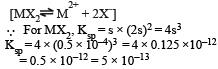QUESTION: 2

### Which has the highest value of pH? 

Solution:

Na2CO3 is a salt of weak acid H2CO3 and strong base NaOH, therefore, its aqueous solution will be basic hence has pH more than 7.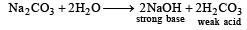QUESTION: 3

### Solution of 0.1 N NH4OH and 0.1 N NH4Cl has pH 9.25. Then find out pKb of NH4OH 

Solution: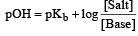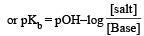but pOH+ pH = 14  or pOH = 14 – pH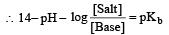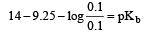14 – 9.25 – 0 = pKb pKb = 4.75

QUESTION: 4

The reaction quotient (Q) for the reaction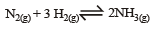is given by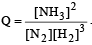The reaction will proceed from right to left if 

where Kc is the equilibrium constant

Solution:

For reaction to proceed from right to left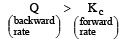i.e the reaction will be fast in backward direction i.e rb > rf.

QUESTION: 5

Which one of the following orders of acid strength is correct? 

Solution:

The higher is the tendency to donate proton, stronger is the acid. Thus the correct order is R – COOH > HOH > R – OH > CH ≡ CH depending upon the rate of donation of proton.

QUESTION: 6

Which one of the following compounds is not a protonic acid? 

Solution:

B(OH)3 does not provide H+ ions in water instead it accepts OH ion and hence it is Lewis acid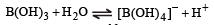QUESTION: 7

The solubility product of AgI at 25ºC is 1.0 × 10–16 mol2 L–2. The solubiliy of AgI in  10–4 N solution of KI at 25ºC is approximately(in mol L–1) 

Solution:

Ksp for AgI = 1 × 10–16 In solution of KI, I would be due to the both AgI and KI, 10–4 solution KI would provide = 10–4 I
AgI would provide, say = x I (x is solubility of AgI)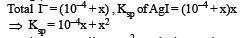as x is very small ∴ x2 can be ignored

∴      10–4 x = 10–16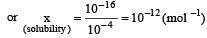QUESTION: 8

The solubility product of a sparingly soluble salt AX2 is 3.2 x 10-11 . Its solubility ( in moles/litre) is


Solution:

For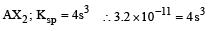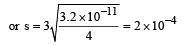QUESTION: 9

The rapid change of pH near the stoichiometric point of an acid-base titration is the basis of indicator detection. pH of the solution is related to ratio of the concentrations of the conjugate acid (HIn) and base (In) forms of the indicator by the expression 

Solution:

For an acid-base indicator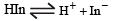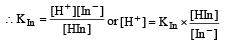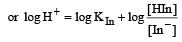Taking negative on both sides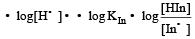or we can write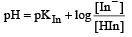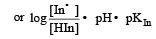QUESTION: 10

H2S gas when passed through a solution of cations containing HCl precipitates the cations of second group of qualitative analysis but not those belonging to the fourth group. It is be cau se 

Solution:

IVth group needs higher S2– i on concentration. In presence of HCl, the dissociation of H2S decreases hence produces less amount of sulphide ions due to common ion effect, thus HCl decreases the solubility of H2S which is sufficient to precipitate IInd group radicals.

QUESTION: 11

What is the correct relation ship between the pHs of isomolar solutions of sodium oxide (pH1), sodium sulphide (pH2), sodium selenide (pH3) and sodium telluride (pH4)? [2 00 5]

Solution:

The solution formed from isomolar solutions of sodium oxide, sodium sulphide, sodium selenide H2O, H2S, H2Se & H2Te respectively.
As the acidic strengh increases from H2O to H2Te thus pH decreases and hence the correct of pHs is pH1 > pH2 > pH3 > pH4.

QUESTION: 12

At 25°C, the dissociation con stant of a base, BOH, is 1. 0 x 10-12. The concentration of hydroxyl ions in 0.01 M aqueous solution of the base would be 

Solution:

Given Kb = 1.0 × 10–12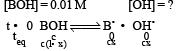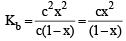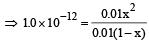On calculation, we get, x = 1.0 × 10–5
Now [OH] =cx
= 0.01 × 10–5
= 1 × 10–7mol L–1

QUESTION: 13

For the reaction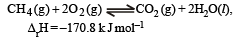Which of the following statements is not true ?

Solution:

First option is incorrect as the value of KP given is wrong. It should have been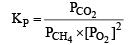QUESTION: 14

The hydrogen ion concentration of a 10–8 M HCl aqueous solution at 298 K (Kw = 10–14) is  

Solution:

For a solution of 10–8 M HCl [H+] = 10–8 [H+] of water = 10–7
Total [H+] = 10–7 + 10–8 = 10 × 10–8 + 10–8
10–8 (10 + 1) = 11 × 10–8

QUESTION: 15

Which of the following pairs constitutes a buffer?

Solution:

HNO2 is a weak acid and NaNO2 is salt of that weak acid and strong base (NaOH).

QUESTION: 16

A weak acid, HA, has a Ka of 1.00 × 10–5. If 0.100 mole of this acid dissolved in one litre of water, the percentage of acid dissociated at equilbrium is closest to 

Solution:

Given Ka = 1.00×10–5,  C= 0.100 mol for a weak electrolyte, degree of dissociation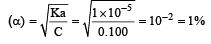QUESTION: 17

The following equilibrium constants are given: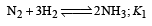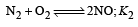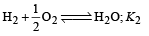The equilibrium constant for the oxidation of NH3 by oxygen to give NO is

Solution:

Given,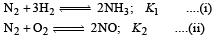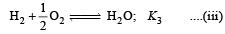We have to calculate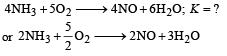For this equation,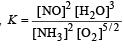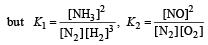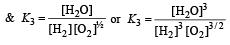Now operate,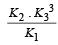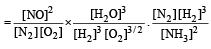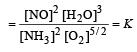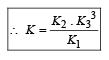QUESTION: 18

Calculate the pOH of a solution at 25°C that contains 1× 10–10 M of hydronium ions, i.e. H3O+.

Solution:

Given [H3O+] = 1 × 10–10 M at 25º  [H3O+] [OH] = 10–14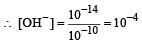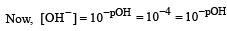∴  pOH = 4

QUESTION: 19

Equal volumes of three acid solutions of pH 3, 4 and 5 are mixed in a vessel. What will be the H+ ion concentration in the mixture ? 

Solution:

[H3O]+ for a solution having pH = 3 is given by [H3O]+ = 1×10–3 moles/litre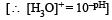Similarly for solution having pH = 4, [H3O]+ = 1 × 10–4 moles/ litre and for pH=5 [H3O+] = 1×10–5 moles/ litre Let the volume of each solution in mixture be IL, then total volume of mixture solution  L = (1 + 1 + 1) L =3L
Total [H3O]+ ion present in mixture solution = (10–3 + 10–4 + 10–5) moles Then [H3O]+ ion concentration of mixture solution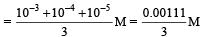=  0.00037 M = 3.7 ×10–4 M.

QUESTION: 20

If the concentration of OH ions in the reaction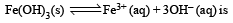decreased by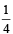times, then equilibriumconcentration of Fe3+ will increase by : 

Solution:

For this reaction Keq. is given by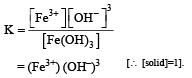If (OH) is decreased by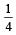times then forreaction equilibrium constant to remain constant, we have to increase the concentration of [Fe3+] by a factor of 43 i.e 4× 4 × = 64. Thus option (c) is correct answer.

QUESTION: 21

Equimolar solutions of the following were prepared in water separately. Which one of the solutions will record the highest pH ? 

Solution:

The highest pH will be recorded by the most basic solution. The basic nature of hydroxides of alkaline earth metals increase as we move from Mg to Ba and thus the solution of BaCl2 in water will be most basic and so it will have  highest pH.

QUESTION: 22

The dissociation equilibrium of a gas AB2 can be represented as :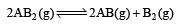The degree of dissociation is ‘x’ and is small compared to 1. The expression relating the degree of dissociation (x) with equilibrium constant Kp and total pressure P is :

Solution:

For the reaction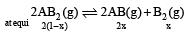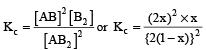=  x3 [(1–x) can be neglected in denominator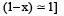The partial pressure at equilibrium are calculated on the basis of total number of moles at equilibrium.
Total number of moles

= 2 (1–x) + 2x + x  = (2 + x)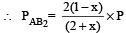where P is the total pressure.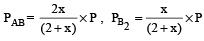Since x is very small so can be neglected in denominator Thus, we get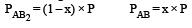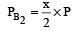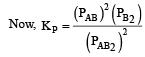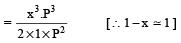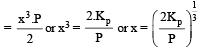QUESTION: 23

The values of Kp1 and Kp2 for the reactions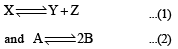are in the ratio of 9 : 1. If degree of dissociation of X and A be equal, then total pressure at equilibrium (1) and (2) are in the ratio :

Solution:

Given reaction are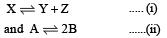Let the total pressure for reaction (i) and (ii) be P1 and P2 respectively, then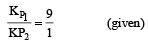After dissociation,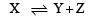At equilibrium (1–α) α α
[Let 1 mole of X dissociate with α as degree of dissociation ]
Total number of moles =   1– α + α + α = (1+α)

Thus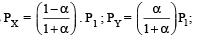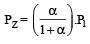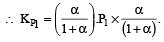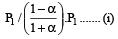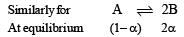We have,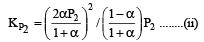Dividing (i) by (ii), we get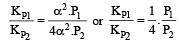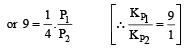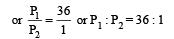i.e. Option (c) is correct answer.

QUESTION: 24

The value of equilibrium constant of the reaction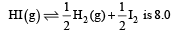The equilibrium constant of the reaction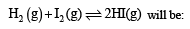Solution:

Given : Equilibrium constant (K1) for the reaction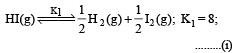To find equilibrium constant for the following reaction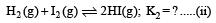For this multiply (i) by 2, we get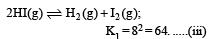[Note: When the equation for an equilibrium is multiplied by a factor, the equilibrium constant must be raised to the power equal to the factor]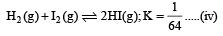Now reverse equation (iii), we get

[Note: For a reversible reaction, the equilibrium constant of the backward reaction is inverse of the equilibrium constant for the forward reaction.] Equation (iv) is the same as the required equation (ii), thus K2 for equation (ii) is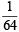i.e.
option (b) is correct.

QUESTION: 25

The dissociation constants for acetic acid and HCN at 25°C are 1.5 × 10–5 and 4.5 × 10–10 respectively. The equilibrium constant for the equilibrium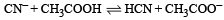would be:

Solution:

Given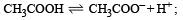Ka1 , = 1.5 × 10– 5 ....(i)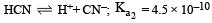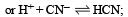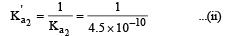∴  From (i) and (ii), we find that the equilibrium constant (Ka) for the reaction ,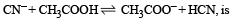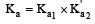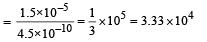QUESTION: 26

Which of the following molecules acts as a Lewis acid ?

Solution:

(CH3)3 B - is an electron deficient, th us behave as a lewis acid.

QUESTION: 27

The ionization constant of ammonium hydroxide is 1.77 × 10–5 at 298 K. Hydrolysis constant of ammonium chloride is: 

Solution:

Ammonium chloride is a salt of weak base and strong acid. In this case hydrolysis constant Kh can be calculated as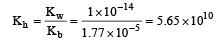QUESTION: 28

If pH of a saturated solution of Ba (OH)2 is 12, the value of its K(sp) is : 

Solution: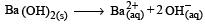pH = 12 or pOH = 2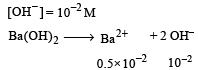[∴ Concentration of Ba 2+ is half of OH-]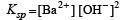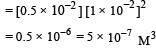QUESTION: 29

What is [H+] in mol/L of a solution that is 0.20 M in CH3COONa and 0.10 M in CH3COOH?Ka for CH3COOH = 1.8 × 10-5 . 

Solution: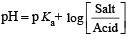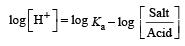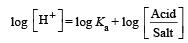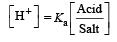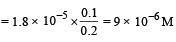QUESTION: 30

In which of the following equilibrium Kc and Kp are not equal? 

Solution: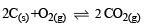Δn = 2 – 1 = + 1

∴ Kc and Kp are not equal.